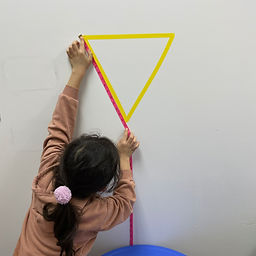Ms. Briana

Target 1​

Lesson Type:

Continuation

Measurment

:

Comparison

Measure and compare objects in standard units.

1:

Understand how to measure length using a standard unit (centimeter, inches).

2:

Understand units of measurement and which unit is most appropriate to measure which attribute.

3:

Measure the length of objects by selecting appropriate measurement tools.

4:

Express, with a unit number, the length difference of objects in terms which is longer or shorter.

5:

Understand that different units will give different measurements of the same object.

4th

Vocabulary:

Ruler, Tape Measure, Yard Stick, Units

Activities:

Students explored the different measuring tools and discussed which was more useful for which purposes.

Students practiced using tools and reading the measurements of different objects using different units.Home Exploration

Guiding Questions:Absent Students:

Target 2

:

1:

Define perimeter.

2:

Understand that perimeter is the distance around a figure.

3:

Understand that perimeter can be found by adding the lengths of the sides of a figure.

4th

Vocabulary:

Perimeter: the distance around the outside of an object/figure.

Activities:

Students used their newly gained knowledge of measuremnt to calculate the perimeter of given shapes in centimeters and inches.Home Exploration

Guiding Questions:Target 3

:

Vocabulary:

Activities:Home Exploration